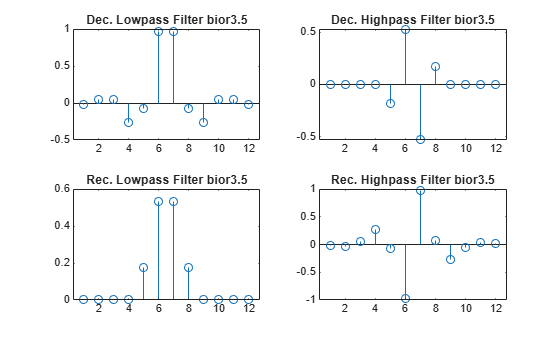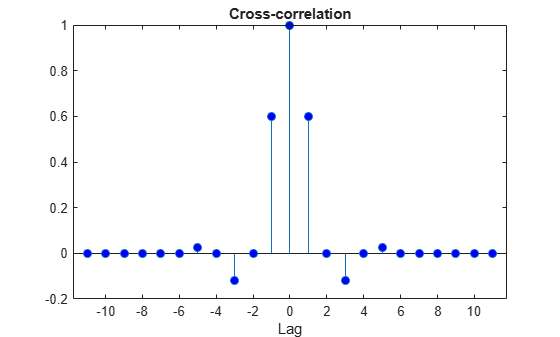# biorfilt

Biorthogonal wavelet filter set

## Syntax

```[Lo_D,Hi_D,Lo_R,Hi_R] = biorfilt(DF,RF) [Lo_D1,Hi_D1,Lo_R1,Hi_R1,Lo_D2,Hi_D2,Lo_R2,Hi_R2] = biorfilt(DF,RF,'8') ```

## Description

The `biorfilt` command returns either four or eight filters associated with biorthogonal wavelets.

`[Lo_D,Hi_D,Lo_R,Hi_R] = biorfilt(DF,RF)` computes four filters associated with the biorthogonal wavelet specified by decomposition filter `DF` and reconstruction filter `RF`. These filters are

 `Lo_D` Decomposition low-pass filter `Hi_D` Decomposition high-pass filter `Lo_R` Reconstruction low-pass filter `Hi_R` Reconstruction high-pass filter

```[Lo_D1,Hi_D1,Lo_R1,Hi_R1,Lo_D2,Hi_D2,Lo_R2,Hi_R2] = biorfilt(DF,RF,'8')``` returns eight filters, the first four associated with the decomposition wavelet, and the last four associated with the reconstruction wavelet.

It is well known in the subband filtering community that if the same FIR filters are used for reconstruction and decomposition, then symmetry and exact reconstruction are incompatible (except with the Haar wavelet). Therefore, with biorthogonal filters, two wavelets are introduced instead of just one:

One wavelet, $\stackrel{˜}{\psi }$, is used in the analysis, and the coefficients of a signal s are

`${\stackrel{˜}{c}}_{j,k}=\int s\left(x\right){\stackrel{˜}{\psi }}_{j,k}\left(x\right)dx$`

The other wavelet, ψ, is used in the synthesis:

`$s=\sum _{j,k}{\stackrel{˜}{c}}_{j,k}{\psi }_{j,k}$`

Furthermore, the two wavelets are related by duality in the following sense:
$\int {\stackrel{˜}{\psi }}_{j,k}\left(x\right){\psi }_{{j}^{\prime },{k}^{\prime }}\left(x\right)dx=0$ as soon as j ≠ j′ or k ≠ k′ and
$\int {\stackrel{˜}{\varphi }}_{0,k}\left(x\right){\varphi }_{0,{k}^{\prime }}\left(x\right)dx=0$ as soon as k ≠ k′.

It becomes apparent, as A. Cohen pointed out in his thesis (p. 110), that “the useful properties for analysis (e.g., oscillations, null moments) can be concentrated in the $\stackrel{˜}{\psi }$ function; whereas, the interesting properties for synthesis (regularity) are assigned to the ψ function. The separation of these two tasks proves very useful.”

$\stackrel{˜}{\psi }$ and ψ can have very different regularity properties, ψ being more regular than $\stackrel{˜}{\psi }$.

The $\stackrel{˜}{\psi }$, ψ, $\stackrel{˜}{\varphi }$ and ϕ functions are zero outside a segment.

## Examples

collapse all

This example shows how to obtain the decomposition (analysis) and reconstruction (synthesis) filters for the `'bior3.5'` wavelet.

Determine the two scaling and wavelet filters associated with the `'bior3.5'` wavelet.

```wv = 'bior3.5'; [Rf,Df] = biorwavf(wv); [LoD,HiD,LoR,HiR] = biorfilt(Df,Rf);```

Plot the filter impulse responses.

```subplot(2,2,1) stem(LoD) title(['Dec. lowpass filter ',wv]) subplot(2,2,2) stem(HiD) title(['Dec. highpass filter ',wv]) subplot(2,2,3) stem(LoR) title(['Rec. lowpass filter ',wv]) subplot(2,2,4) stem(HiR) title(['Rec. highpass filter ',wv])```Demonstrate that autocorrelations at even lags are only zero for dual pairs of filters. Examine the autocorrelation sequence for the lowpass decomposition filter.

```npad = 2*length(LoD)-1; LoDxcr = fftshift(ifft(abs(fft(LoD,npad)).^2)); lags = -floor(npad/2):floor(npad/2); figure stem(lags,LoDxcr,'markerfacecolor',[0 0 1]) set(gca,'xtick',-10:2:10)```Examine the cross correlation sequence for the lowpass decomposition and synthesis filters. Compare the result with the preceding figure.

```npad = 2*length(LoD)-1; xcr = fftshift(ifft(fft(LoD,npad).*conj(fft(LoR,npad)))); lags = -floor(npad/2):floor(npad/2); stem(lags,xcr,'markerfacecolor',[0 0 1]) set(gca,'xtick',-10:2:10)```Compare the transfer functions of the analysis and synthesis scaling and wavelet filters

```dftLoD = fft(LoD,64); dftLoD = dftLoD(1:length(dftLoD)/2+1); dftHiD= fft(HiD,64); dftHiD = dftHiD(1:length(dftHiD)/2+1); dftLoR = fft(LoR,64); dftLoR = dftLoR(1:length(dftLoR)/2+1); dftHiR = fft(HiR,64); dftHiR = dftHiR(1:length(dftHiR)/2+1); df = (2*pi)/64; freqvec = 0:df:pi; subplot(2,1,1) plot(freqvec,abs(dftLoD),freqvec,abs(dftHiD),'r') axis tight title('Transfer modulus for dec. filters') subplot(2,1,2) plot(freqvec,abs(dftLoR),freqvec,abs(dftHiR),'r') axis tight title('Transfer modulus for rec. filters')```## References

Cohen, A. (1992), “Ondelettes, analyses multirésolution et traitement numérique du signal,” Ph. D. Thesis, University of Paris IX, DAUPHINE.

Daubechies, I. (1992), Ten lectures on wavelets, CBMS-NSF conference series in applied mathematics. SIAM Ed.